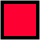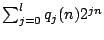# Centre for Discrete and Applicable Mathematics## CDAM Research Report, LSE-CDAM-2004-16

November 2004

Nicholas Georgiou

Abstract

Let Tn be the complete binary tree of height n, with root 1n as the maximum element. For T a tree, define A(n;T) to be the number of subtrees S of Tn, with S isomorphic to T and 1n in S, and define C(n;T) to be the total number of subtrees S of Tn with S isomorphic to T. We disprove a conjecture of Kubicki, Lehel and Morayne, which claims that A(n;T1)/C(n;T1) ≤ A(n;T2)/C(n;T2) for any fixed n and arbitrary rooted trees T1, T2 with T1 a subtree of T2 . We show that A(n;T) is of the formwhere l is the number of leaves of T, and each qj is a polynomial. We provide an algorithm for calculating the two leading terms of ql for any tree T. We investigate the asymptotic behaviour of the ratio A(n;T)/C(n;T) and give examples of classes of pairs of trees T1, T2 where it is possible to compare A(n;T1)/C(n;T1) and A(n;T2)/C(n;T2). By calculating these ratios for a particular class of pairs of trees, we show that the conjecture fails for these trees, for all sufficiently large n. Kubicki, Lehel and Morayne have proved the conjecture when T1, T2 are restricted to being binary trees. We also look at embeddings into other complete trees, and we show how the result can be viewed as one of many possible correlation inequalities for embeddings of binary trees. We also show that if we consider strict order-preserving maps of T1, T2 into Tn (rather than embeddings) then the corresponding correlation inequalities for these maps also generalise to arbitrary trees.

A PDF file (268 kB) with the full contents of this report can be downloaded by clicking here.

Alternatively, if you would like to get a free hard copy of this report, please send the number of this report, LSE-CDAM-2004-16, together with your name and postal address to:CDAM Research Reports Series Centre for Discrete and Applicable Mathematics London School of Economics Houghton Street London WC2A 2AE, U.K.Phone: +44(0)-20-7955 7732. Fax: +44(0)-20-7955 6877. Email: info@cdam.lse.ac.uk